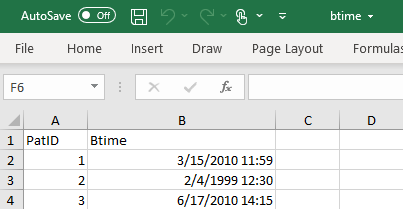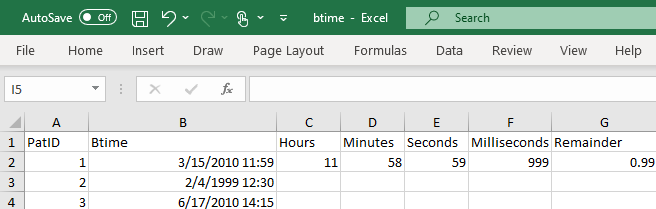»  Home »  Resources & support »  FAQs »  Why does my Excel datetime value seem to be behind in Stata?

## Why does my Excel datetime value seem to be behind in Stata?

 Title Why does my Excel datetime value seem to be behind in Stata? Author Kevin Crow, StataCorp Gabriela Ortiz, StataCorp

The import excel command will properly convert Microsoft Excel’s numerically encoded date and times to Stata’s numerically encoded date and times. However, in some cases, the time value may seem to be behind in Stata. Why is this?

The short answer is that Excel rounds its datetime values to the nearest millisecond, while Stata doesn’t. A difference of less than a millisecond, when rounded, could result in values that look as if they’re off by a whole minute. Depending on the date and time, this slight difference could carry over to the day or even year. Below, we dive into the details and show you how to match the datetimes displayed by Excel.

### How Excel and Stata handle datetimes

One important thing to understand is that Stata and Excel store their datetimes differently. Stata stores datetimes as the number of milliseconds elapsed since January 1, 1960 00:00:00.000. Excel stores date and time values together in a single number. Datetimes on or after 01mar1900 00:00:00 are stored as days plus fraction of day since 30dec1899 00:00:00, such as ddddddd.tttttttt. The integer records the days, and the fractional part records the number of seconds from 00:00:00, the beginning of the day, divided by the number of seconds in 24 hours (24x60x60 = 86,400).

For example, I have an Excel file with fictional data on patients and their time of birth. It seems patient 1 was born on March 15, 2010, at 11:59 a.m.Let’s import these times into Stata.

. import excel "btime.xlsx", firstrow
(2 vars, 3 obs)

. list



PatID             Btime
1.      1   3/15/2010 11:58
2.      2    2/4/1999 12:30
3.      3    6/17/2010 2:15



In Stata, that first time appears as 11:58, so it seems to be a minute off from the time we saw in Excel. Let’s change the formatting so we can see the milliseconds.

. format Btime %tcnn/dd/ccYY_hh:MM.SS.sss

. list



PatID                    Btime
1.      1   3/15/2010 11:58.59.999
2.      2    2/4/1999 12:30.00.000
3.      3    6/17/2010 2:15.00.000



When we compare this to the time in Excel, it seems we’re only off by a millisecond. To see what is going on, we’ll take a closer look at how Excel is rounding its datetime values. We’ll first compute the hour of the datetime value using Excel functions as follows:

MOD(INT(0.tttttttt*24), 24)


Here 0.tttttttt refers to the fractional portion of a 24-hour day. Multiplying this fraction by 24 gives us the number of hours into the day. In terms of our worksheet, because our datetime is stored in cell B2, we’ll compute this as

MOD(INT((B2-INT(B2))*24), 24)


where B2-INT(B2) gives us the fractional portion of the day because we’re subtracting the integer portion (date) of the datetime value. For example, if we’re looking at 12 p.m., this would be a value of 0.5 (12/24=0.5). We multiply this by 24 and then use INT to round down to the nearest integer to get the number of hours. The MOD() function will divide the first number by the second and give us the remainder.

We’ll use these functions in the same manner to compute the minutes, seconds, milliseconds, and the remainder:

minutes      = MOD(INT((B2-INT(B2))*24*60), 60)
seconds      = MOD(INT((B2-INT(B2))*24*60*60), 60)
milliseconds = MOD(INT((B2-INT(B2))*24*60*60*1000), 1000)
remainder    = MOD(INT((B2-INT(B2))*24*60*60*1000*100), 100)/100


There are 24x60 minutes in a day, 24x60x60 seconds in a day, and so on. We’ve entered these formulas into cells C2-G2 in our Excel worksheet:So the first birth time in Excel is really during the 58th minute, not the 59th. But Excel is rounding the 0.99 to the nearest millisecond, which then adds on to the 59th second, and finally on to the 58th minute, making it 59. And the time of birth we saw in Stata was correct; it was 11:58. In this case, our dates in Stata seemed to be a minute off. But imagine if our datetime value was instead December 31, 2019, 23:59:59.999, a fraction of a millisecond from the end of the year. If we were to round up the fraction of a millisecond to a millisecond, the millisecond would carry over to the day and thus the year.

Stata doesn’t round datetime values; 59.999 seconds after 23:59 p.m. is not quite midnight. And if you choose to display datetime information at a lower resolution, Stata will truncate the values. So if you choose to display your times at the minute resolution, you will see 23:59.

### Matching datetimes displayed by Excel

If you want to match the datetimes displayed by Excel, you can use Stata's round() function to round to the nearest millisecond

. replace Btime = round(Btime, 1)
(2 real changes made)

. list



PatID                    Btime
1.      1   3/15/2010 11:59.00.000
2.      2    2/4/1999 12:30.00.000
3.      3    6/17/2010 2:15.00.000



Or you can round to the nearest second:

. replace Btime = round(Btime, 1000)